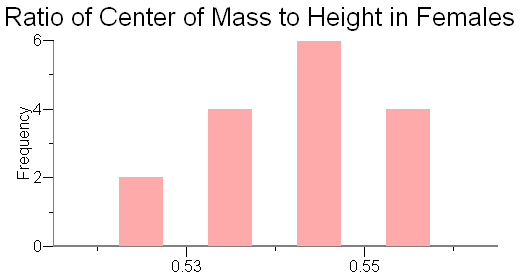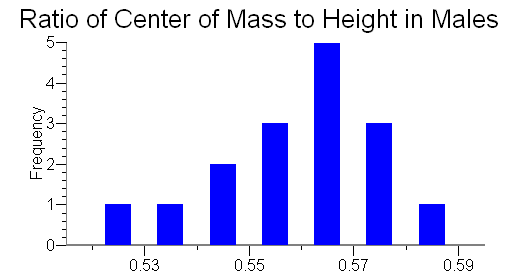# Center of Mass of a Human

An educational, fair use website

## Abstract

The purpose of this experiment is to locate the center of mass of people, to determine whether the center of mass is different for males and females, and to calculate the ratio of a person's center of mass to his/her height.

## Introduction

The center of mass is the balance point of an object's mass. If a pivot were placed at this point, the object would remain in place and be balanced. The center of mass of a system is not always at the geometric center of the system. For example, a car's center of mass is closer to the ground rather than in the geometric center of the car so that the car is better balanced. Another example of this is the technique of a high jumper. A high jumper bends his body in a certain way so that the center of mass does not clear the bar, but the body does.

When a system is balanced around its center of mass, it is said to be in a state of equilibrium. The center of mass can be referred to as a pivot point around which the system can revolve. The system revolves due to the rotational equivalents of force, known as torques, which rotate the system either clockwise or counterclockwise. Placing a pivot at the center of mass of a system results in that system being in equilibrium and having a net torque of zero. On each end of a long, rigid body, the torque on one end is equal in magnitude but opposite in direction to the torque on the other end, resulting in a net torque of zero. The formula for torque is:

Στ = rF

Where r = radius and F = force. It is possible to locate the center of mass of a system by placing a pivot at the theoretical center of mass and using the formula for torque by setting the torques on either end of a long, rigid body equal to each other.

## Diagram## Procedure

1. Lay two 2 × 4 wooden beams of approximately 243 cm (8 ft) length side by side across two scales so that the ends of each beam are situated at the center of each scale.
2. Attach two meter sticks end to end on one side of one of the beams. This is done in order to measure one's height when a person is lying down.
3. Record the weight of the beams without anyone on top of them. This is the tare weight.
4. Have a person remove his/her shoes, and then lay across the beams, making sure his/her heels line up with the end of one side of the beams. Have the person lay with their toes pointing upward and hands at their sides.
5. Record the weight on each scale. If the scales are not in the same units, use a conversion to make the data have common units, i.e. kilograms to pounds, pounds to kilograms.
6. Record the height of the person using the meter sticks that are attached to one of the wooden beams.

## Analysis

In order to determine the center of mass of a person, we used the formula for torque:

Στ = rF

The torques due to the weight on each end of the person were set equal to each other. The displayed weight on the scale was the force, and there are two different radii. The first radius is the distance to the center of mass from the person's feet, and the second radius is the length of the board minus the distance to the center of mass from the person's feet. The net torque of the system is zero and therefore the torques on the opposite sides of the boards must be equal:

w1x = w2(l-x),

where w1 is equal to the weight at the person's feet, x is equal to the distance from the person's feet to his/her center of mass, w2is equal to the weight at the head, and l is equal to the length of the beams. The resulting formula, when solved for the distance to the center of mass from the person's feet (radius one) is:

x = w2l/(w1 + w2)

After determining the location of each person's center of mass, the ratio of the center of mass to the height of each person was calculated using the formula:

x/h,

where x is the location of the person's center of mass and h is the person's height.Figure 1: This histogram shows the frequency of the different Ratio of Center of Mass to Height values obtained from the female test subjects. (Data for Female Sampling)Figure 2: This histogram shows the frequency of the different Ratio of Center of Mass to Height values obtained from the male test subjects. (Data for Male Sampling)

## Conclusion

1. A person's center of mass is slightly below his/her belly button, which is nearly the geometric center of a person.
2. Males and females have different centers of mass- females' centers of mass are lower than those of males.
3. The average ratio of center of mass to height in females is approximately 0.543 and the average ratio of center of mass to height in males is approximately 0.560.

## Sources of Error

1. Clothing contributed to the weight of the subjects and therefore resulted in a shift in each person's center of mass.
2. The subjects' heartbeats caused their center of mass to shift because one's center of mass changes as the heart dilates and constricts- pumping blood throughout the body.

Stephanie Gambino, Michael Mirochnik, Scott Schechter -- 2005

Students Choice